Digital Logic | Latches

Latches are basic storage elements that operate with signal levels (rather than signal transitions). Latches controlled by a clock transition are flip-flops. Latches are edge-sensitive devices. Latches are useful for the design of the asynchronous sequential circuit.

SR (Set-Reset) Latch – SR Latch is a circuit with:
(i) 2 cross-coupled NOR gate or 2 cross-coupled NAND gate.
(ii) 2 input S for SET and R for RESET.
(iii) 2 output Q, Q’.

Q Q’ STATE
1 0 Set
0 1 Reset

Under normal conditions, both the input remains 0. The following is the RS Latch with NAND gates: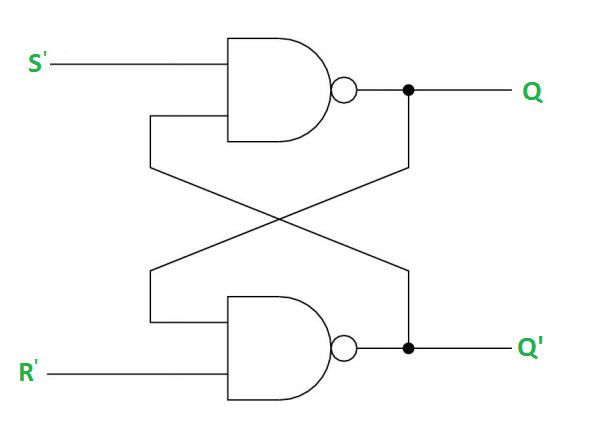Case-1: S’=R’=1 (S=R=0) –
If Q = 1, Q and R’ inputs for 2nd NAND gate are both 1.
If Q = 0, Q and R’ inputs for 2nd NAND gate are 0 and 1 respectively.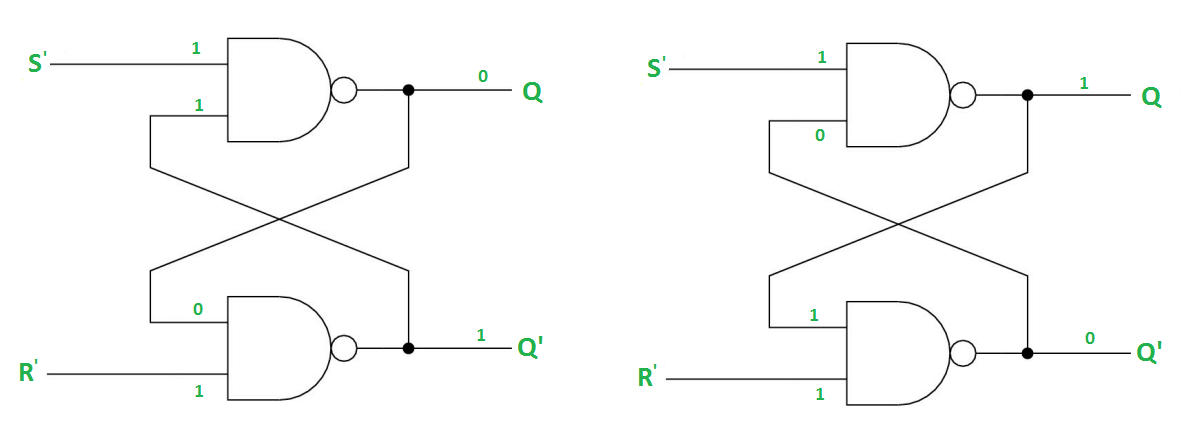Case-2: S’=0, R’=1 (S=1, R=0) –
As S’=0, the output of 1st NAND gate, Q = 1(SET state). In 2nd NAND gate, as Q and R’ inputs are 1, Q’=0.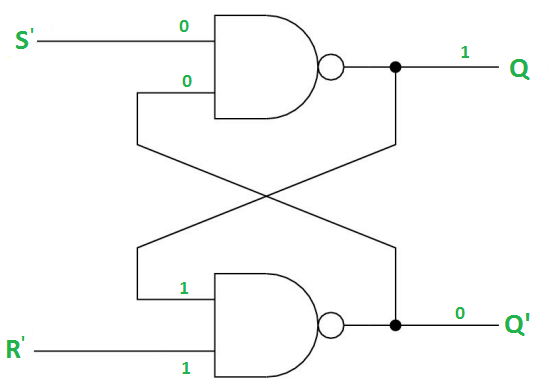Case-3: S’= 1, R’= 0 (S=0, R=1) –
As R’=0, the output of 2nd NAND gate, Q’ = 1. In 1st NAND gate, as Q and S’ inputs are 1, Q=0(RESET state).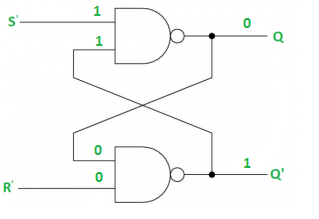Case-4: S’= R’= 0 (S=R=1) –
When S=R=1, both Q and Q’ becomes 1 which is not allowed. So, the input condition is prohibited.

The SR Latch using NOR gate is shown below: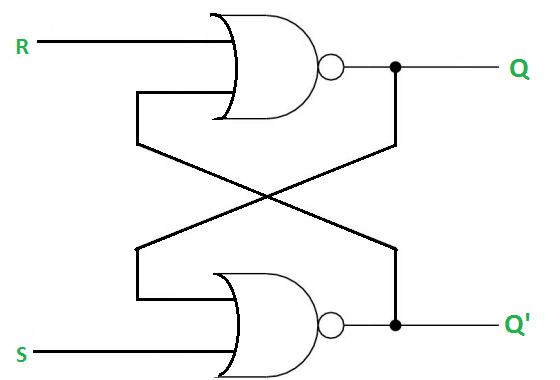Gated SR Latch –
A Gated SR latch is a SR latch with enable input which works when enable is 1 and retain the previous state when enable is 0.Gated D Latch –
D latch is similar to SR latch with some modifications made. Here, the inputs are complements of each other. The design of D latch with Enable signal is given below: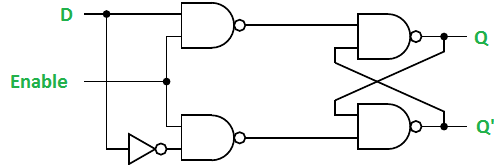The truth table for the D-Latch is shown below:

Enable D Q(n) Q(n+1) STATE
1 0 x 0 RESET
1 1 x 1 SET
0 x x Q(n) No Change

As the output is same as the input D, D latch is also called as Transparent Latch. Considering the truth table, the characteristic equation for D latch with enable input can be given as:

Q(n+1) = EN.D + EN'.Q(n)

Reference:
DIGITAL ELECTRONICS – Atul P. Godse, Mrs. Deepali A. GodseMy Personal Notes arrow_drop_upCheck out this Author's contributed articles.

If you like GeeksforGeeks and would like to contribute, you can also write an article using contribute.geeksforgeeks.org or mail your article to contribute@geeksforgeeks.org. See your article appearing on the GeeksforGeeks main page and help other Geeks.

Please Improve this article if you find anything incorrect by clicking on the "Improve Article" button below.